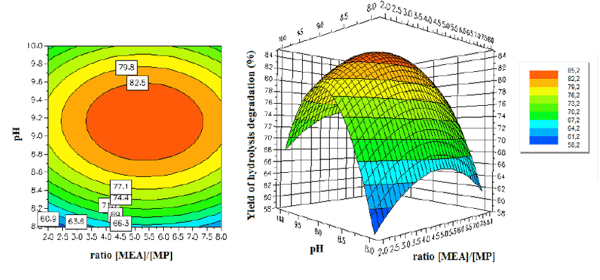Related Tags

contour plot
matplotlib
python

# How to draw a contour plot in matplotlibEducative Answers Team

## What are contour plots?

To visualize the relationship between three ​variables, we generally need a three-dimensional graph. A contour plot is a 2D diagram that uses circles (often colored) to represent the third axis. Any point on the ​circle has the same value in the third axis.Matplotlib makes it fairly simple to draw contour plots.

## Steps

### 1. Import libraries

We don’t need to import the entire matplotlib module, pyplot should be enough. Also, import numpy for any mathematics needed for the plot.

from matplotlib import pyplot as plt
import numpy as np #For mathematics, and making arrays

### 2. Create a panel


width_of_panel = 4
height_of_panel = 3
d = 1000
plt.figure(figsize=(width_of_panel, height_of_panel), dpi=d)


### 3. Introduce the data

This usually comprises of two independent-variable arrays and a dependent variable array (the contour).


x = np.arange(0, 25, 1)
y = np.arange(0, 25, 1)
x, y = np.meshgrid(x, y)
z = np.sin(x/3) + np.cos(y/4)


### 4. Draw the contour plot

plt.contour(x, y, z)

### 5. Make the contour plot

Now, let’s combine all of this to make a simple contour plot.

import matplotlib.pyplot as plt
import numpy as np

width_of_panel = 4
height_of_panel = 3
d = 1000
plt.figure(figsize=(width_of_panel, height_of_panel), dpi=d)
x = np.arange(0, 25, 1)
y = np.arange(0, 25, 1)
x, y = np.meshgrid(x, y)
z = np.sin(x/3) + np.cos(y/4)#np.sin(x * np.pi / 2) + np.cos(y * np.pi / 3)

plt.contour(x, y, z)

### 6. Fill in the contour plot

To make a filled contour plot, just replace contour with contourf.

import matplotlib.pyplot as plt
import numpy as np

width_of_panel = 4
height_of_panel = 3
d = 1000
plt.figure(figsize=(width_of_panel, height_of_panel), dpi=d)
x = np.arange(0, 25, 1)
y = np.arange(0, 25, 1)
x, y = np.meshgrid(x, y)
z = np.sin(x/3) + np.cos(y/4)#np.sin(x * np.pi / 2) + np.cos(y * np.pi / 3)

plt.contourf(x, y, z)

RELATED TAGS

contour plot
matplotlib
python